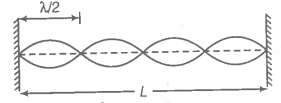Show that when a string fixed at its two ends vibrates in 1 loop, 2 loops, 3 loops and 4 loops, the frequencies are in the ratio 1:2:3:4.

Hint: Use the derivation of standing waves.
Step 1: Find the frequency of n-harmonic.
Let, there are n numbers of loops in the string.Length corresponding each loop is $\frac{\mathrm{\lambda }}{2}$.
Now, we can write,